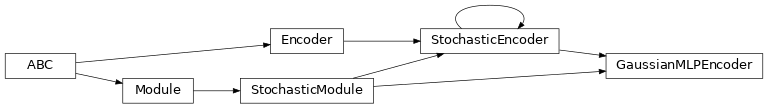# garage.tf.embeddings.gaussian_mlp_encoder¶

GaussianMLPEncoder.

class GaussianMLPEncoder(embedding_spec, name='GaussianMLPEncoder', hidden_sizes=(32, 32), hidden_nonlinearity=tf.nn.tanh, hidden_w_init=tf.initializers.glorot_uniform(seed=deterministic.get_tf_seed_stream()), hidden_b_init=tf.zeros_initializer(), output_nonlinearity=None, output_w_init=tf.initializers.glorot_uniform(seed=deterministic.get_tf_seed_stream()), output_b_init=tf.zeros_initializer(), learn_std=True, adaptive_std=False, std_share_network=False, init_std=1.0, min_std=1e-06, max_std=None, std_hidden_sizes=(32, 32), std_hidden_nonlinearity=tf.nn.tanh, std_output_nonlinearity=None, std_parameterization='exp', layer_normalization=False)GaussianMLPEncoder with GaussianMLPModel.

An embedding that contains a MLP to make prediction based on a gaussian distribution.

Parameters
• embedding_spec (garage.InOutSpec) – Encoder specification.

• name (str) – Model name, also the variable scope.

• hidden_sizes (list[int]) – Output dimension of dense layer(s) for the MLP for mean. For example, (32, 32) means the MLP consists of two hidden layers, each with 32 hidden units.

• hidden_nonlinearity (callable) – Activation function for intermediate dense layer(s). It should return a tf.Tensor. Set it to None to maintain a linear activation.

• hidden_w_init (callable) – Initializer function for the weight of intermediate dense layer(s). The function should return a tf.Tensor.

• hidden_b_init (callable) – Initializer function for the bias of intermediate dense layer(s). The function should return a tf.Tensor.

• output_nonlinearity (callable) – Activation function for output dense layer. It should return a tf.Tensor. Set it to None to maintain a linear activation.

• output_w_init (callable) – Initializer function for the weight of output dense layer(s). The function should return a tf.Tensor.

• output_b_init (callable) – Initializer function for the bias of output dense layer(s). The function should return a tf.Tensor.

• learn_std (bool) – Is std trainable.

• adaptive_std (bool) – Is std a neural network. If False, it will be a parameter.

• std_share_network (bool) – Boolean for whether mean and std share the same network.

• init_std (float) – Initial value for std.

• std_hidden_sizes (list[int]) – Output dimension of dense layer(s) for the MLP for std. For example, (32, 32) means the MLP consists of two hidden layers, each with 32 hidden units.

• min_std (float) – If not None, the std is at least the value of min_std, to avoid numerical issues.

• max_std (float) – If not None, the std is at most the value of max_std, to avoid numerical issues.

• std_hidden_nonlinearity (callable) – Nonlinearity for each hidden layer in the std network. It should return a tf.Tensor. Set it to None to maintain a linear activation.

• std_output_nonlinearity (callable) – Nonlinearity for output layer in the std network. It should return a tf.Tensor. Set it to None to maintain a linear activation.

• std_parameterization (str) –

How the std should be parametrized. There are a few options: - exp: the logarithm of the std will be stored, and applied a

exponential transformation

• softplus: the std will be computed as log(1+exp(x))

• layer_normalization (bool) – Bool for using layer normalization or not.

build(self, embedding_input, name=None)

Build encoder.

Parameters
• embedding_input (tf.Tensor) – Embedding input.

• name (str) – Name of the model, which is also the name scope.

Returns

Distribution. tf.tensor: Mean. tf.Tensor: Log of standard deviation.

Return type

tfp.distributions.MultivariateNormalDiag

property spec(self)

garage.InOutSpec: Specification of input and output.

property input_dim(self)

int: Dimension of the encoder input.

property output_dim(self)

int: Dimension of the encoder output (embedding).

property vectorized(self)

bool: If this module supports vectorization input.

get_latent(self, input_value)

Get a sample of embedding for the given input.

Parameters

input_value (numpy.ndarray) – Tensor to encode.

Returns

An embedding sampled from embedding distribution. dict: Embedding distribution information.

Return type

numpy.ndarray

Note

It returns an embedding and a dict, with keys - mean (numpy.ndarray): Mean of the distribution. - log_std (numpy.ndarray): Log standard deviation of the

distribution.

get_latents(self, input_values)

Get samples of embedding for the given inputs.

Parameters

input_values (numpy.ndarray) – Tensors to encode.

Returns

Embeddings sampled from embedding distribution. dict: Embedding distribution information.

Return type

numpy.ndarray

Note

It returns an embedding and a dict, with keys - mean (list[numpy.ndarray]): Means of the distribution. - log_std (list[numpy.ndarray]): Log standard deviations of the

distribution.

property distribution(self)

Encoder distribution.

Returns

Encoder distribution.

Return type

tfp.Distribution.MultivariateNormalDiag

property input(self)

tf.Tensor: Input to encoder network.

property latent_mean(self)

tf.Tensor: Predicted mean of a Gaussian distribution.

property latent_std_param(self)

tf.Tensor: Predicted std of a Gaussian distribution.

clone(self, name)

Return a clone of the encoder.

It copies the configuration of the primitive and also the parameters.

Parameters

name (str) – Name of the newly created encoder. It has to be different from source encoder if cloned under the same computational graph.

Returns

Newly cloned encoder.

Return type

garage.tf.embeddings.encoder.Encoder

reset(self, do_resets=None)

Reset the encoder.

This is effective only to recurrent encoder. do_resets is effective only to vectoried encoder.

For a vectorized encoder, do_resets is an array of boolean indicating which internal states to be reset. The length of do_resets should be equal to the length of inputs.

Parameters

do_resets (numpy.ndarray) – Bool array indicating which states to be reset.

property name(self)

str: Name of this module.

property state_info_specs(self)

State info specification.

Returns

keys and shapes for the information related to the

module’s state when taking an action.

Return type

List[str]

property state_info_keys(self)

State info keys.

Returns

keys for the information related to the module’s state

when taking an input.

Return type

List[str]

terminate(self)

Clean up operation.

get_trainable_vars(self)

Get trainable variables.

Returns

A list of trainable variables in the current

variable scope.

Return type

List[tf.Variable]

get_global_vars(self)

Get global variables.

Returns

A list of global variables in the current

variable scope.

Return type

List[tf.Variable]

get_regularizable_vars(self)

Get all network weight variables in the current scope.

Returns

A list of network weight variables in the

current variable scope.

Return type

List[tf.Variable]

get_params(self)

Get the trainable variables.

Returns

A list of trainable variables in the current

variable scope.

Return type

List[tf.Variable]

get_param_shapes(self)

Get parameter shapes.

Returns

A list of variable shapes.

Return type

List[tuple]

get_param_values(self)

Get param values.

Returns

Values of the parameters evaluated in

the current session

Return type

np.ndarray

set_param_values(self, param_values)

Set param values.

Parameters

param_values (np.ndarray) – A numpy array of parameter values.

flat_to_params(self, flattened_params)

Unflatten tensors according to their respective shapes.

Parameters

flattened_params (np.ndarray) – A numpy array of flattened params.

Returns

A list of parameters reshaped to the

shapes specified.

Return type

List[np.ndarray]